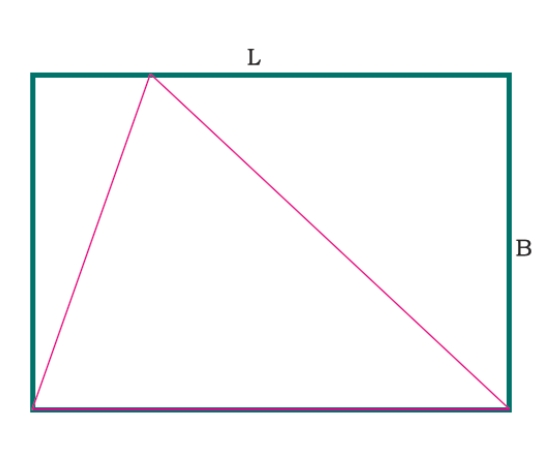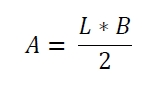# Area of largest triangle that can be inscribed within a rectangle in C Program?

Suppose one rectangle is given. We know the length L and breadth B of it. We have to find the area of largest triangle that can be inscribed within that rectangle −The largest triangle will always be the half of the rectangle. So it will be## Example

#include <iostream>
#include <cmath>
using namespace std;
float area(float l, float b) {
if (l < 0 || b < 0 ) //if the valuse are negative it is invalid
return -1;
float area = (l*b)/2;
return area;
}
int main() {
float a = 10, b = 8;
cout << "Area : " << area(a, b);
}

## Output

Area : 40